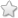# TNSB - 8 - Maths :: Algebra

## Course Content / Subtopic list:

1. Recall Addition And Subtraction Of Expressions.
2. Multiply Algebraic Expressions With Integer Coefficient
3. Divide Algebraic Expressions By Monomials.
4. The Algebraic Identities
5. Expressions That Can Be Factorize
6. Word Problems That Involve Linear Equations

## Lesson Plan

[Contributor - Ananth]

Dear Volunteer,

Please find below the detailed lesson plan and PPT for this chapter in the language mentioned against the respective link. While the lesson plan will give an overall flow of the lesson, the PPT will include lesson flow as well as the presentations for the class.

You may use the content as it is or download and modify them to suit the students requirements.

If you feel any major modifications or additions are carried out by you in the form of adding any new activity, presentations, videos etc. we request you to send the modified version to submitcontent@evidyloka.org for enabling upload the modified version.

The worksheets of the lesson can be accessed from the worksheet tab.

Kindly rate the lesson plan and share your suggestions and comments on the lesson plan in the comments box below. Your valuble suggestions and comments will help us to improve the content.

## Videos

[Contributor - Sumathi Sampath]

https://www.youtube.com/watch?v=pUrbcWA1bxY (Multiply two monomials with two variables)

## Images

[Contributor - Kripa S]

https://www.mathsisfun.com/algebra/images/variable-constant.gif (variable, terms)

https://i.ytimg.com/vi/hK_LQaNDxi4/hqdefault.jpg (examples of equation)

https://userscontent2.emaze.com/images/814e43c0-30ed-4335-b6ac-d428ffac5544/daf7e0213128c586230e1c613e8581c0.png (example of variable in equation)

http://tutorial.math.lamar.edu/Classes/Alg/Polynomials_files/eq0002MP.gif (degree of expression)

https://i.ytimg.com/vi/zat2KIJ-mFA/maxresdefault.jpg (value of expression)

http://media1.shmoop.com/images/algebra-ii/alg2_rational_graphik_3.png (how to frame expressions)

## Activities

[Contributor - Kripa S]

1.Quiz: I have some chocolates with me. I gave 4 chocolates to my sister. I have 17 chocolates left. How many did I have in the beginning? Make an equation.

2. How many pens/ sticks you would need to make alphabet A? How many sticks you would need to make 10 As ? Repeat this rule for alphabets E, H, V, I

3. Play in pairs. One student ask the other to think of a number. Multiply the number by 2, then add 2, multiply 5, then add 5, multiply 10, then add 10. Ask the friend for answer. You can guess what the number is. Trick is ignore the units and ten's place digits. Consider the left digits, subtract 1 and that is the number thought by the student. Make an equation of this game

[Contributor - Preetha Sridharan]

[Contributor - Ananth]

Lesson Plan version 1.1 PPThttps://drive.google.com/open?id=1ivM5bw0nho789Zz3JmLw1IDnJ2HcpZ7H (PPT - English)

## Worksheets

[Contributor - Preetha Sridharan]

Worksheets [Version 2021]

Worksheets [Version 2021]

## Assessments

[Contributor - Prasanna Oruganti](0 votes)